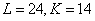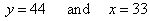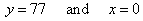Economic interpretation of calculus operations - multivariate

SHOW SOLUTIONS

Practice problems

The following is a quiz on your understanding of multivariate rates of change and optimization techniques.  After you work through the problems, you can link to a step by step solution to each problem.1a.b.c.d.2a.b.c.d.3a.b.c.d.[Index]